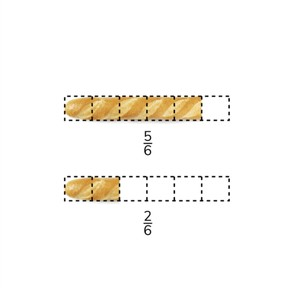Converting fractions greater than 1 to mixed numbers after adding

# Converting fractions greater than 1 to mixed numbers after adding

I can convert fractions greater than 1 to mixed numbers after addition.8,000 schools use Gynzy92,000 teachers use Gynzy1,600,000 students use Gynzy

## General

Students learn to add fractions with common denominators and add to fractions greater than 1, and learn to convert them to mixed numbers.

## Common core standard(s)

CCSS.MATH.CONTENT.4.NF.B.3.C

## Relevance

Discuss with students that it is useful to learn how to add fractions with a common denominator and learn to add to a fraction greater than 1 so you can determine how much you have in total.

## Introduction

Show students a fraction and ask them to simplify it as much as possible. Erase the grey box to reveal the answer.

## Development

Check that students understand converting fractions greater than 1 to mixed numbers after adding by asking the following question:
- What do you do if you want to add fractions with a common denominator?

## Guided practice

Students first are asked to add fractions with common denominators with visual support. Students then solve without visual support and finally are given fraction story problems to solve.

## Closing

Discuss the importance of being able to convert from fractions greater than 1 to mixed numbers after adding with your students. That will allow you to add fractions easily. On the interactive whiteboard, show a number of fractions that students must add. Each addition problem is linked to an image that they must drag to the correct answer. If all images are placed correctly, a completed picture is made.

## Teaching tips

Students who have difficulty adding fractions with common denominators can first practice adding fractions that don't become greater than 1. Then they can practice adding to fractions greater than 1 and converting to mixed numbers.

### The online teaching platform for interactive whiteboards and displays in schools

• Save time building lessons

• Manage the classroom more efficiently

• Increase student engagement